# Numerical Study of Mixed Convection in Cylinrical Czochralski Configuration for Crystal Growth of Silicon

Numerical Study of Mixed Convection in Cylinrical Czochralski Configuration for Crystal Growth of Silicon

Atia Aissa Bouabdallah Said Teggar Mohamed Benchatti Ahmed

Mechanical Laboratory, University of Laghouat, Laghouat 03000, Algeria

Page:
39-46
|
DOI:
https://doi.org/10.18280/ijht.330106
N/A
|
Accepted:
N/A
|
Published:
31 March 2015
| Citation

OPEN ACCESS

Abstract:

A numerical study of the mixed convection of liquid metal in Czochralski configuration for crystal growth was carried out. The finite volume method with SIMPLER Algorithm was used to solve the conservation equations of mass, momentum and energy. Due to computational constraints, the simulations were limited to axisymmetric geometries. The  obtained  results  are compared to the experimental data of the literature. The effect of Richardson number on the flow structure and the  thermal field has been presented and discussed for Ri = 0.1, 0.5, 1,  1.5 and 2.  The effect  of the aspect  ratio of the cavity  was also analysed. The results showed that increasing of Richardson number increases the velocity of the fluid in the cavity and reduces the rate of heat transfer. The aspect ratio had a significant effect on the velocity and thermal fields.

Keywords:

Mixed convection, liquid metal, Czochralski technique, finite volume method.

1. Introduction

The single crystals production is an important issue for the optoelectronics industry and electronics . Most commercial single crystals of semiconductors are grown from melt. This is mainly due to the simplicity of the melt growth systems, and also because of high growth rates achieved with this technique. The most used melt growth techniques are Czochralski (Cz), Bridgman (B) and zone-freezing (ZF). Today, more than 80% of bulk commercial crystals are grown by the Czochralski technique. This technique has been particularly successful in growth of single crystals of silicon (Si), germanium (Ge) and compounds such as gallium arsenide (GaAs).

The fluid flow in the Cz melt plays a significant role on the quality of the crystal , It is governed by a number of interacting forces such as buoyancy, Coriolis, centrifugal forces and surface tension . Several researchers were interested in the study of mixed convection caused by crystal and/or crucible rotation during crystal growth, the studies were carried under different conditions with the addition of several factors such as magnetic field [4-7], and radiation  in order to improve the quality of the obtained single crystals. Basu et al.  have found that the crystal rotation affects the quality and the stability of the growing crystal, the centrifugal force due to the rotating crystal leads to a stabilizing effect on the three dimensional flow caused by natural convection. They have found a critical value of Richardson number (Ri = Gr/Re2 = 235) beyond it the flow become periodic. Galazka and Wilke  have showed that during the growth of YAG, the interface convexity decreases when the crystal rotation rate increases, it becomes almost flat for high Reynolds number values. Crochet et al.  studied numerically the effect of forced convection induced by rotation on the Czochralski crystal growth of GaAs in a cylindrical crucible for different Reynolds number values. Liu and Kakimoto  analyzed the effect of crystal rotation on the growth interface shape in the presence of a transverse magnetic field, they found that the melt-crystal interface changes from an obvious 3D shape when the crystal is not rotating to an almost 2D shape with increase in rotation rate. Mahfouz and Badr  treated the problem of the mixed convection of a horizontal cylinder carrying out rotating oscillatory movement in a fluid at rest of infinite dimension. Gelfgat et al.  presented a detailed numerical study of stable conditions and the onset of oscillatory instabilities of axisymmetric rotating Newtonian incompressible fluid flow confined in a vertical cylinder, with two disks rotating independently at the top and the bottom. Turbulent natural convection of a heat generating low Prandtl number fluid is studied numerically in a cylindrical enclosure. Computations are carried out for three different liquid metals . Xinjun  reported numerical simulations of the Navier–Stokes equations for the axisymmetric recirculating zones during spin-up and spin-down for confined rotating fluid flows. Iwatsu  examined numerically the heat transfer characteristics of rotating viscous, incompressible and axisymmetric fluid flow generated by the constant rotation of the top disk in a cylindrical enclosure of aspect ratio Ar = 1. Hirata  found that unstable flow components and forced convection due to the rotating crucible bottom were electively suppressed and stable forced convection flows were produced. He also showed that due to the change in the melt motion, the radial uniformity was increased, temperature fluctuations within the melt were significantly decreased, and the radial temperature gradient was increased. Valentine and Jahnke  examined the flow field inside a cylindrical enclosure induced by the rotation of the top and bottom end walls with a fixed sidewall: a stable oscillatory solution was found for a Reynolds number Re = 3×103 and for aspect ratio γ = 1.5. A numerical study of the periodic flow for γ = 2.5 was conducted by Lopez and Perry . They showed the existence of oscillatory modes.

The objective of the present paper is to carry out a numerical study of mixed convection in a cylindrical Czochralski configuration filled with silicon liquid. We study the effect of Richardson number and the aspect ratio on the velocity and thermal fields. We presented the results in terms of stream functions, isotherms, velocities and average Nusselt numbers.

2. Mathematical Formulation

The problem under consideration consists of a vertical cylindrical crucible (Fig.1) of H height and RC radius with an aspect ratio Ar = H/RC. The crucible is completely filled with a silicon liquid Pr = 0.011﴿. The melt is bounded above partially by a crystal of radius Rs (Rs = RC/3), rotating at angular velocity S and by a flat free surface. The crystal surface is cooled by TC (TC > melting temperature) and the sidewall of the crucible is maintained at higher temperature Th (Th > TC) whereas the bottom surface is assumed to be adiabatic. The thermo-physical properties of Silicon used in the present study are given in Table .1.

The following governing equations were written in non-dimensional forms by using RC , Ω.RC , ρ(ΩRC)2, (Th-TC) and ν.RC respectively, as the scale factors for the length, velocity, pressure, temperature and stream function. The governing equations are obtained by taking into account the following assumptions:

- Laminar flow, stationary and axisymmetric with swirl.

- The physical properties of the fluid are assumed constant, and the Boussinesq approximation is valid.

- The liquid metal is incompressible and Newtonian.

- Joule heating and viscous dissipation are negligible.

- The free surface and the crystal-melt interface are assumed to be flat.

• Continuity equation

$\frac{1}{R} \frac{\partial}{\partial R}(R U)+\frac{\partial V}{\partial Z}=0$     (1)

• Momentum equation in R-direction

$\frac{1}{R} \frac{\partial}{\partial R}\left(R U^{2}\right)+\frac{\partial}{\partial Z}(V U)-\frac{W^{2}}{R}= -\frac{\partial P}{\partial R}+\frac{1}{R e}\left[\frac{1}{R} \frac{\partial}{\partial R}\left(R \frac{\partial U}{\partial R}\right)+\frac{\partial^{2} U}{\partial Z^{2}}-\frac{U}{R^{2}}\right]$     (2)

• Momentum equation in Ө-direction

$\frac{1}{R} \frac{\partial}{\partial R}(R U W)+\frac{\partial}{\partial Z}(V W)+\frac{U W}{R}= \frac{1}{\operatorname{Re}}\left[\frac{1}{R} \frac{\partial}{\partial R}\left(R \frac{\partial W}{\partial R}\right)+\frac{\partial^{2} W}{\partial Z^{2}}-\frac{W}{R^{2}}\right]$      (3)

• Momentum equation in Z-direction

$\frac{1}{R} \frac{\partial}{\partial R}(R U V)+\frac{\partial}{\partial Z}\left(V^{2}\right)= -\frac{\partial P}{\partial Z}+\frac{1}{R e}\left[\frac{1}{R} \frac{\partial}{\partial R}\left(R \frac{\partial V}{\partial R}\right)+\frac{\partial^{2} V}{\partial Z^{2}}\right]+R i \cdot \theta$   (4)

• Energy equation

$\frac{1}{R} \frac{\partial}{\partial R}(R U \theta)+\frac{\partial}{\partial Z}(V \theta)= \frac{1}{\operatorname{Re} \cdot \operatorname{Pr}}\left[\frac{1}{R} \frac{\partial}{\partial R}\left(R \frac{\partial \theta}{\partial R}\right)+\left(\frac{\partial^{2} \theta}{\partial Z^{2}}\right)\right]$    (5)

The boundary conditions are given as:

Along the bottom wall (Z = 0, 0 < R <1);

U = V = W = 0, $\frac{\partial \theta}{\partial Z}$ = 0      (6a)

Along the crystal-melt interface (Z = Ar; 0 < R < RS);

U = V = 0, W = R, $\theta$ = 0     (6b)

Along the free surface (Z = Ar; RS < R < 1);

$\frac{\partial U}{\partial Z}$ = V = W = 0, $\frac{\partial \theta}{\partial Z}$ = 0       (6c)

Along the side walls (0 < Z < Ar; R = 1);

U = V = W = 0, $\theta$ = 1        (6d)

Along the symmetry axe (0 < Z < Ar; R = 0);

U = W = 0, $\frac{\partial V}{\partial R}$= 0, $\frac{\partial \theta}{\partial R}$= 0      (6e)

## 1.png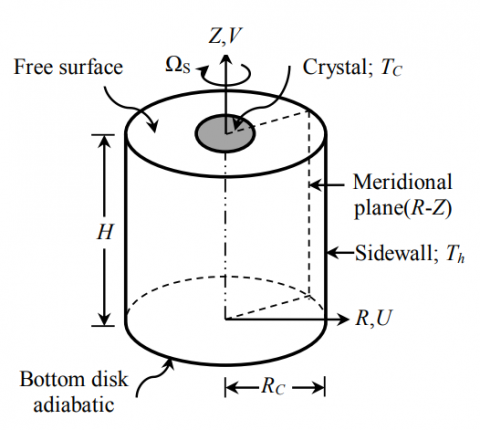Figure 1. Sketch of physical problem with boundary conditions.

3. Numerical Method

The governing equations (Eqs.1-5) with the associated boundary conditions (Eqs.6a-e) are solved using a finite volume method. Scalar quantities (P, W and) are stored at the center of control volume, whereas the vectorial quantities (U, V) are stored on the faces of each volume. For the discretisation of spatial terms, a second-order central difference scheme is used for the diffusion and convection parts of the mathematical model, and the SIMPLER Algorithm  is used to determine the pressure from continuity equation. The obtained algebraic equations are solved by the line-by-line tri-diagonal matrix algorithm (TDMA).The convergence is obtained when the maximum relative change between two consecutive iteration levels is less than 10-4. Calculations are carried out on a PC with CPU 3 GHz.

The increments $\Delta R$ and $\Delta Z$ of the grid are not uniform, they are chosen according to geometric progressions of ration equal to 1.05, which permitted grid refinement near the walls. In order to examine the effect of the grid size on the numerical solution, a number of sizes have been investigated for grid independence: 45×90, 60×120, 75×150 and 90×180 nodes. The results are illustrated in (Figs.2a-b), examination of these curves allowed us to conclude that 75×150 and 90×180 give better information on the nature of the flow and these results have guided the choice of the final mesh of 75×150. As the program execution time for the grid 90×180 is greater than the chosen grid. This choice is considered to show the best compromise between computational time and precision.

Table 1. Thermophysical properties of Si melt

 Physical property Symbol Value Unit Density ρ 2570 [kg.m-3] Dynamic viscosity µ 7.75×10-4 [kg.s-1.m-1] Kinematics viscosity ν 3.01×10-7 [m2.s-1] Conductivity λ 66.9 [W.m-1.K-1] Specific heat Cp 915 [J.kg-1.K-1] Thermal diffusivity α 2.845×10-5 [m2.s-1] Thermal expansion β 1.41×10-4 [K-1] Prandtl number Pr 0.011 [-]
4. Results and Discussion

4.1 Validation of the computer code

Some comparisons with experimental results available in the literature are realized. Firstly, a comparison is made for the distribution of the radial and swirl velocity (U, W) along the vertical line (R = 0.60) with the experimental data (Figs. 3a-b) obtained by Michelson . The author used the LDA technique (Laser Doppler Anemometry) to determine the distribution of radial velocity in cylindrical cavity, the upper disk is rotating and that for Re = 1800 and Ar = 1. The computed values are in fair agreement with experimental measurements. Secondly, a comparison was made with the experimental results due to Karcher et al. . They used a cylindrical cavity of aspect ratio Ar = 4.125, filled with a fluid of low Prandtl number (Pr = 0.0203), the bottom and lateral wall were cooled by circulation of the water, and the upper wall heated electrically. The distribution of the radial velocity component U along R = 0.25 is showed in Fig.4. Our numerical results are in good agreement with the experimental results except for the region Z = (0, 0.6), where we can observe a slight difference between the two results.

## 2a.png(a)

## 2b.png(b)

Figure 2. Profiles of dimensionless axial velocity component V (a), and radial velocity component U (b), in the middle of the cavity for various grids

## 3a.png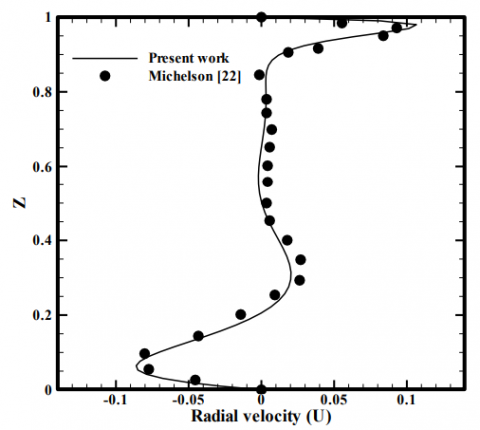(a)

## 3b.png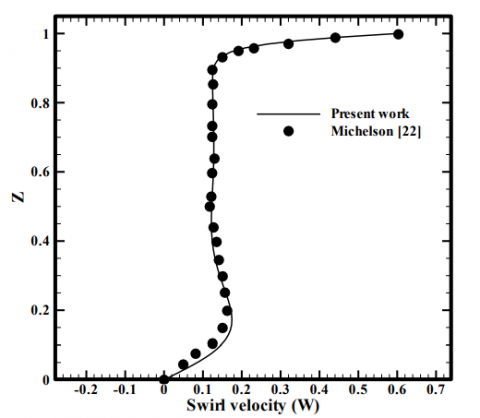(b)

Figure 3. Comparison of the present results with experimental measurements of Michelson ; Profiles of radial velocity component U (a), and swirl velocity component W (b) along R = 0.60

## 4.png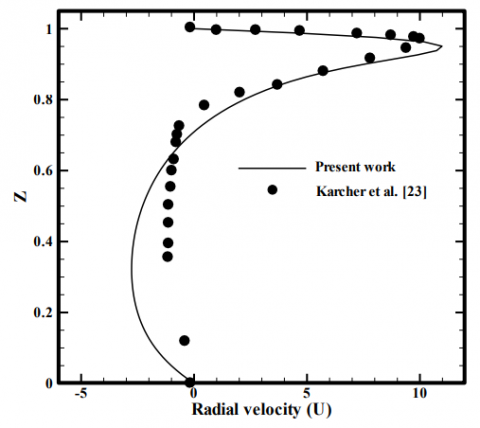Figure 4. Comparison of the present results with experimental measurements of Karcher et al. ; Profiles of radial velocity component U along the R = 0.25

4.2Effect of the Richardson number

To study the effect of Richardson number in the velocity and thermal field, we set the Reynolds number at Re = 1500, aspect ratio at Ar = 2 and we varied the Richardson number for following values: Ri = 0.1, 0.5, 1, 1.5 and 2.  In Figs. 5-7, we have presented the streamlines, isothermal lines and the contours of the azimuthal velocity component for various values of the Richardson number.

For low Richardson number values (Ri < 1), we see that the flow is unicellular, the main cell rotates counterclockwise created by the rotating crystal on one side and on the other hand supplement the effect of buoyant forces acting from top to bottom which generates increased velocity in a significant way, that the convection mode present in the case is natural convection. For Ri = 1, we see the birth of a second vortex that rotates clockwise, this vortex due to two types of convection, natural and forced convection, same vortex found by Wua et al. . For Ri > 1 the forced convection dominate, the anticlockwise vortex becomes bigger and more intense and decomposed due to the acceleration of particles of liquid metal in the heart of the cavity.

Fig. 6, shows the temperature distribution in the cavity.It is found that the heat exchange occurs but with a dominance of conductive regime especially in the region under crystal where the isotherms become noticeably compressed horizontally (a strong gradient), and a remarkable deviation of occurs in the insulated region of the contacts of the fluid at the side wall. This deviation is directed towards the hot side wall. When we increase the number of Richardson, the isotherms become distorted. We can connect this deformation to the increases of velocity (Figs.8a, b), this creates a high intensity rate of flow which promotes convective appearance.

The variation of the temperature according to Z at R = 0 for various values of the Richardson number is illustrated in Fig.9a, we find a high temperature gradient near the crystal and the side wall, which results in these areas better heat exchange, on the contrary in the middle of the enclosure exchange is low.The variation of average Nusselt number in function of the Richardson number is shown in Fig. 9b. We can see that average Nusselt decreases with increasing of the Richardson number, this allows us to conclude that the heat transfer improves as much as Ri decreased.

4.3Effect of the aspect ratio

In this section, we study the effect of aspect ratio Ar = H/ RC (H, RC are the height and radius of the cavity respectively) in the flow and heat transfer. We set the Reynolds number at Re = 1500 and Richardson number at Ri = 0.5, the cavity filled by metal liquid (Silicon, Pr = 0.011) and subject to the same temperature gradient. Four aspect ratios Ar = 1/3, 1/2, 1, 2 and 3 were tested (Figs. 10a-e).

It is noted that the flow is unicellular, for all cavities, these cells circulate in the counterclockwise, except to the aspect ratio Ar = 3 where the flow is bicellular. We note that the streamline are inclined and extend according to the extension of the cavity. This means that the walls of the cavity effects on the structure of movement of fluid particles. For isothermal line, we can see that they are almost parallel to the cold wall, which means that the intense heat transfer in this region.

The average Nusselt number is plotted as a function of aspect ratio (Ar) in Fig.12, it can be seen that the average Nusselt number is an increasing function of the aspect ratio Ar of the cavity as the most effective configuration is where the vertical exchange surface (active walls) is large (Fig.11b), since the maximum value of average Nusselt number is in the maximum configuration of large heat exchange surface (Ar = 3). This is in agreement with the numerical results of Turan and Poole .

The Profile of axial velocity component along Z = 1 is presented in Fig. 11a, we can see that the variation of aspect ratio has an important effect on the velocity field where the increase of this factor stabilizes the velocity in the cavity and the best stabilization found in large aspect ratio (Ar = 3). Similar results were found by Oudina and Bessaih .

## 5.png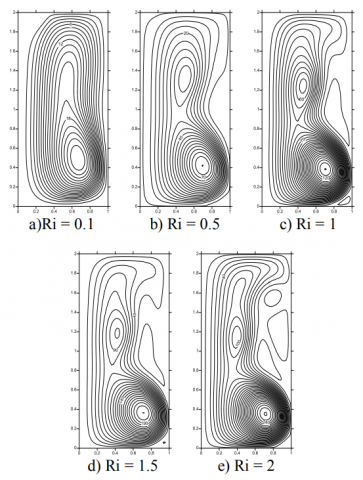Figure 5. Streamline for various values of the Richardson number

## 6a.png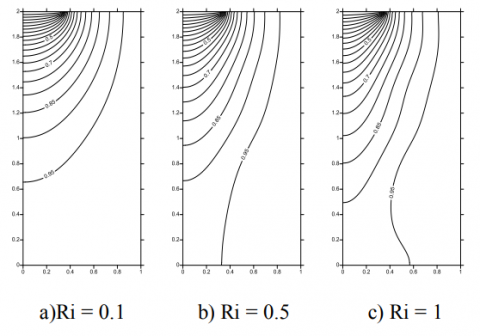## 6b.png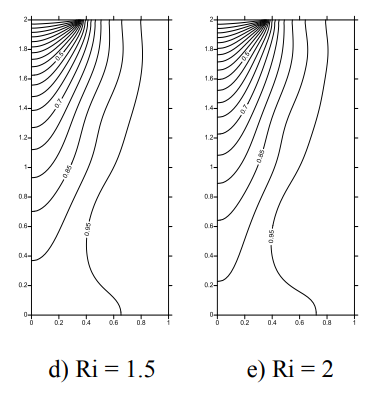Figure 6. Isothermal lines for various values of the Richardson number

## 7a.png## 7b.png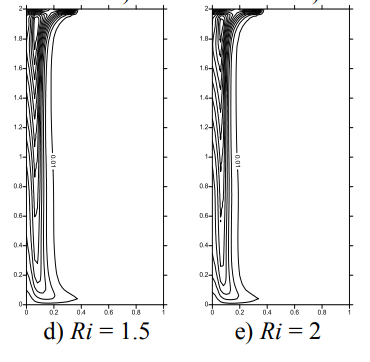Figure 7. Contours of azimuthal velocity component for various values of the Richardson number

## 8a.png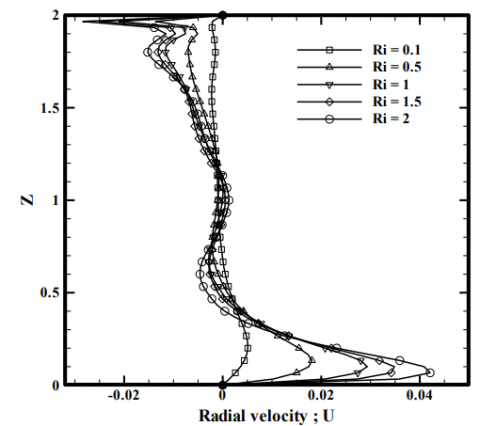(a)

## 8b.png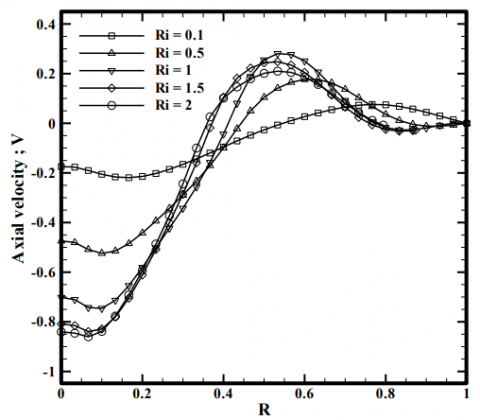(b)

Figure 8. Profiles of radial velocity component U along of R = 0 (a), and axial velocity component V along of Z = 1 (b), for various values of the Richardson number

## 9a.png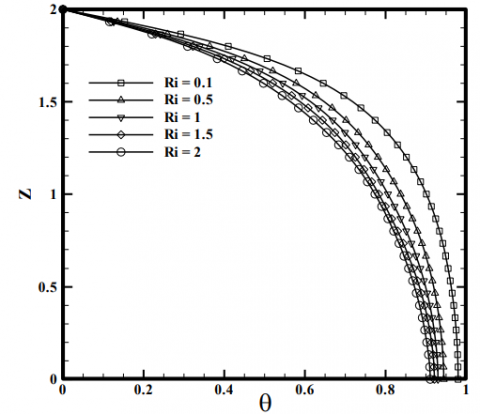(a)

## 9b.png(b)

Figure 9. Profiles of temperature Ө along of R = 0(a), and average Nusselt number for various values of the Richardson number (b)

## 10a.pnga)  Ar = 1/3

## 10b.png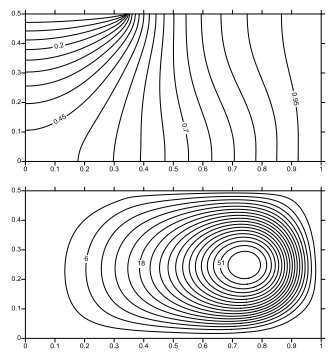b)  Ar =1/2

## 10c.png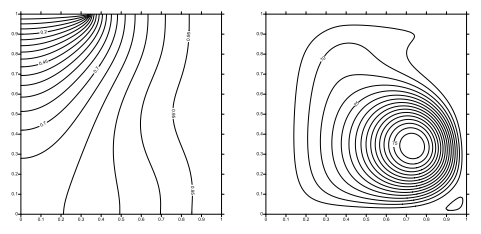c)  Ar = 1

## 10d.png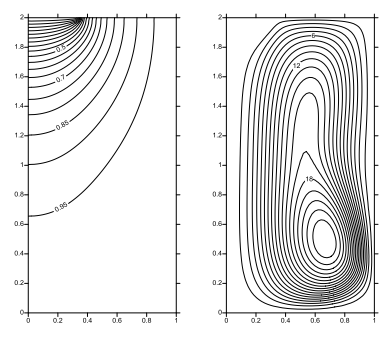d)  Ar = 2

## 10e.png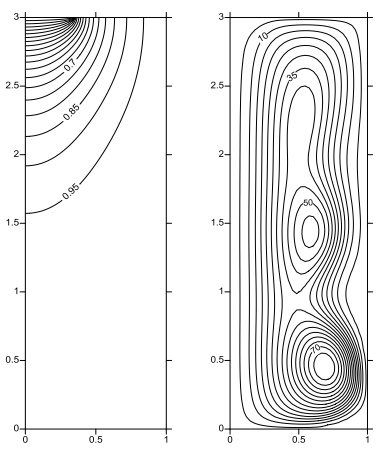e)  Ar = 3

Figure 10. Isothermal ligne (right) and stream function (left), for various aspect ratios

## 11a.png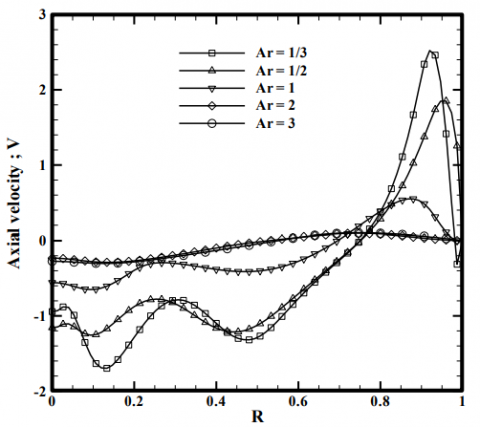(a)

## 11b.png(b)

Figure 11. Profile of axial velocity component V along of   Z = 1(a), and temperature Ө along of R = 0 (b), for various aspect ratios

## 12.png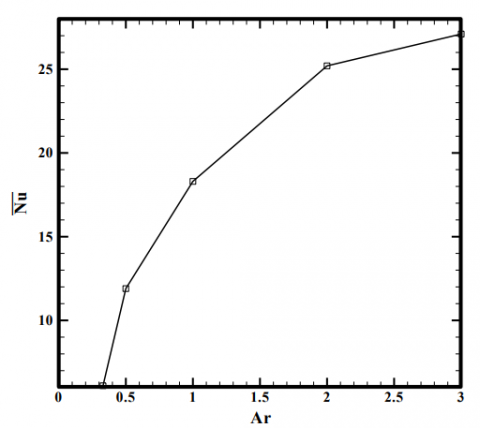Figure 12. Average Nusselt number for various aspect ratios

5. Conclusion

A numerical study of mixed convection in the cylindrical Czochralski configuration for crystal growth of the silicon, the finite volume method has been used to solve the mathematical model. The main results obtained in this study can be summarized as follows:

• The computer code developed in this study was validated with the results found in the literature, and good agreement has been obtained.

• Increasing of the Richardson number increases the velocity of the fluid in cavity and reduces the rate of heat transfer.

• The strongest stabilization of the velocity field occurs in the low Richardson number.

• The change of aspect ratio has a significant effect on the velocity field and heat transfer, where the best stabilization found in a large aspect ratio.

The results presented in this work, allowing experimenters by the Czochralski method, the preparation of their processes of semiconductor in good conditions, in order to improve the quality of the semiconductors obtained during the crystal growth.

Nomenclature
 Latin symbols Ar Aspect ratio Cp Specific heat (J. kg-1. K-1) g Gravitational acceleration (m. s-2) H Height of the cylinder (m) Nu Average Nusselt number P Dimensionless pressure Rc Radius of the cylinder (m) R, Z Dimensionless meridional coordinates T Temperature (K) U Dimensionless radial velocity V Dimensionless axial velocity W Dimensionless azimutal velocity Dimensionless numbers Pr Prandtl number Re Reynlolds number Ri Richardson number Greek symbols α Thermal diffusivity (m2. s-1) β Thermal expansion coefficient (K-1) λ Thermal conductivity (W. m-1. K-1) Ө Dimensionless temperature υ Kinematic viscosity (m2. s-1) ρ Density of the fluid (kg. m-3) Ψ Dimensionless stream function Ω Angular velocity (rad. s-1) Subscripts c cold h hot
References

1.    D.T. J. Hurle, Convective Transport in Melt Growth Systems, J. Crystal Growth, vol. 65, pp. 124-132, 1983.

2.    S.M. Pimputkar and S. Ostrach, Convective Effects in Crystals Grown from Melt, J. Crystal Growth, vol. 55, pp. 614-646, 1981.

3.    W.E. Langlois, Buoyancy-driven Flows in Crystal Growth Melts, Ann. Rev. Fluid Mech., vol.17, pp. 191- 215, 1985.

4.    Y. Collet, O. Magotte, N.V. Bogaert, R. Rolinsky, F. Loix a, M. Jacot, V. Regnier, J. Marchal and F. Dupret, Effective Simulation of the Effect of a Transverse Magnetic Field (TMF) in Czochralski Silicon Growth, J. Crystal Growth, vol. 360, pp. 18-24, 2012.

5.    X. Cen, Y.S. Li and J. Zhan, Three Dimensional Simulation of Melt Flow in Czochralski Crystal Growth with Steady Magnetic Fields, J. Crystal Growth, vol. 340, pp. 135-141, 2012.

6.    X. Chen, J. Zhan, Y.S. Li and X. Cen, Large Eddy Simulation of Industrial Czochralski Si Crystal Growth under Transverse Magnetic Field, J. Crystal Growth, vol. 389, pp. 60-67, 2014.

7.    F. G. Shehadeh and H. M. Duwairi , MHD Natural Convection with Joule and Viscous heating Effect in Porous Media-filled Enclosures, Int. J. Heat and Technology, vol. 28 (1), pp. 31-36, 2010.

8.    E.M. Nunes, M.H.N. Naraghi, H. Zhang and V. Prasad, A Volume Radiation Heat Transfer Model for Czochralski Crystal Growth Processes, J. Crystal Growth, vol. 236, pp. 596-608, 2002.

9.    B. Basu, S. Enger, G. Brenner and F. Durst, Effect of Crystal Rotation on the Three-dimensional Mixed Convection in the Oxide Melt for Czochralski Growth , J. Crystal Growth, Vol. 230 no.1-2, pp. 148-154, 2001.

10.    Z. Galazka and H. Wilke, Influence of Marangoni Convection on the Flow Pattern in the Melt During Growth of Y3Al5O12 Single Crystals by  The Czochralski Method, J. Crystal Growth, Vol. 216 no.1-4, pp. 389-398, 2000.

11.    M. J. Crochet, P. J. Wouters, F. T. Geyling and A. S. Jordan, Finite Element Simulation of Czochralski Bulk Flow, J. Crystal Growth, vol. 65 no. 1-3, pp. 153-165, 1983.

12.    L. Liu and K. Kakimoto, 3D Global Analysis of CZ–Si Growth in a Transverse Magnetic Field with Various Crystal Growth Rates, J. Crystal Growth, vol. 275, pp. 1521-1526, 2005.

13.    F.M. Mahfouz and H.M. Badr, Heat Convection from a Cylinder Performing Steady Rotation or Rotary Oscillation. Part II: Rotary oscillation, Heat and Mass Transer, vol. 34 no. 5, pp. 375- 380, 1999.

14.    A. K. Sharma, K. Velusamy and C. Balaji, Turbulent Natural Convection of Heat Generating Low Prandtl Fluids in a Cylindrical Enclosure, Int. J. Heat and Technology, vol. 28 (1), pp. 9-17, 2010.

15.    A.Y. Gelfgat, P.Z. Bar-Yoseph and A. Solan, Steady States and Oscillatory Instability of Swirling Flow in a Cylinder with Rotating Top and Bottom, Phys. Fluids , vol. 8, pp. 2614-2625, 1996.

16.    C. Xinjun, A Numerical Study of the Recirculation Zones During Spin-up and Spindown for Confined Rotating Flows, Theor. Comput. Fluid Dyn., vol. 17, pp. 31-49, 2003.

17.    R. Iwatsu, Flow Pattern and Heat Transfer of Swirling Flows in Cylindrical Container with Rotating Top and Stable Temperature Gradient, Int. J. Heat Mass Transf., vol. 47, pp. 2755-2767, 2004.

18.    H. Hirata and K. Hoshikawa, Three Dimensional Numerical Analyses of the Effects of a Cusp Magnetic Field on the Flows, Oxygen  Transport  and  Heat Transfer in a Czochralski Silicon Melt, J. Crystal Growth, vol. 125 no. 1-2, pp. 181-207, 1992.

19.    T. Valentine and C.C. Jahnke, Flows Induced in a Cylinder with Both end Walls Rotating, Phys. Fluids, vol. 6, pp. 2702-2710, 1994.

20.    J.M. Lopez and A.D. Perry, Axisymmetric Vortex Breakdown. Part 3: Onset of periodic flow and chaotic advection, J. Fluid Mech., vol. 234, pp. 449-471, 1992.

21.    S.V. Patankar, Numerical Heat Transfer and Fluid Flow, Hemisphere. Washington, D. C., 1980.